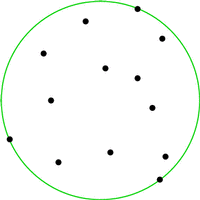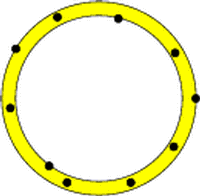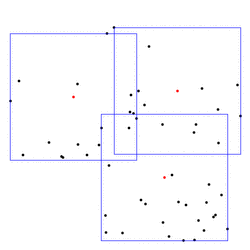# Chapter 62Bounding Volumes

Kaspar Fischer, Bernd Gärtner, Thomas Herrmann, Michael Hoffmann, and Sven SchönherrThis chapter describes algorithms which for a given point set compute the ``best'' circumscribing object from a specific class. If the class consists of all spheres in d-dimensional Euclidean space and ``best'' is defined as having smallest radius, then we obtain the smallest enclosing sphere problem already mentioned above.

In the following example a smallest enclosing circle (CGAL::Min_circle_2<Traits>) is constructed from points on a line and written to standard output. The example shows that it is advisable to switch on random shuffling in order to deal with a `bad' order of the input points.

```File: examples/Min_circle_2/min_circle_2.cpp
```
```#include <CGAL/Exact_predicates_exact_constructions_kernel.h>
#include <CGAL/Min_circle_2.h>
#include <CGAL/Min_circle_2_traits_2.h>
#include <iostream>

// typedefs
typedef  CGAL::Exact_predicates_exact_constructions_kernel K;
typedef  CGAL::Min_circle_2_traits_2<K>  Traits;
typedef  CGAL::Min_circle_2<Traits>      Min_circle;

typedef  K::Point_2                      Point;

int
main( int, char**)
{
const int n = 100;
Point P[n];

for ( int i = 0; i < n; ++i)
P[ i] = Point( (i%2 == 0 ? i : -i), 0);
// (0,0), (-1,0), (2,0), (-3,0), ...

Min_circle  mc1( P, P+n, false);    // very slow
Min_circle  mc2( P, P+n, true);     // fast

CGAL::set_pretty_mode( std::cout);
std::cout << mc2;

return 0;
}
```

Other classes for which we provide solutions are ellipses (CGAL::Min_ellipse_2<Traits>), rectangles (CGAL::min_rectangle_2), parallelograms (CGAL::min_parallelogram_2) and strips (CGAL::min_strip_2) in the plane, with appropriate optimality criteria. For arbitrary dimensions we provide smallest enclosing spheres for points (CGAL::Min_sphere_d<Traits>) and spheres for spheres (CGAL::Min_sphere_of_spheres_d<Traits>), smallest enclosing annuli (CGAL::Min_annulus_d<Traits>), and approximate minimum-volume enclosing ellipsoid with user-specified approximation ratio (CGAL::Approximate_min_ellipsoid_d<Traits>).Bounding volumes can be used to obtain simple approximations of complicated objects. For example, consider the problem of deciding whether two moving polygons currently intersect. An obvious solution is to discretize time and perform a full intersection test for any time step. If the polygons are far apart most of the time, this is unnecessary. Instead, simple bounding volumes (for examples, circles) are computed for both polygons at their initial positions. At subsequent time steps, an intersection test between the moving bounding circles replaces the actual intersection test; only if the circles do intersect, the expensive intersection test between the polygons is performed. In practice, bounding volume hierarchies are often used on top of simple bounding volumes to approximate complicated objects more accurately.

Bounding volumes are also frequently applied to extract geometric properties of objects. For example, the smallest enclosing annulus of a point set can be used to test whether a set of points is approximately cospherical. Here, the width of the annulus (or its area, or still another criterion that we use) is a good measure for this property. The largest area triangle is for example used in heuristics for matching archaeological aerial photographs. Largest perimeter triangles are used in scoring cross country soaring flights, where the goal is basically to fly as far as possible, but still return to the departure airfield. To score simply based on the total distance flown is not a good measure, since circling in thermals allows to increase it easily.

Bounding volumes also define geometric ``center points'' of objects. For example, if two objects are to be matched (approximately), one approch is to first apply the translation that maps the centers of their smallest enclosing spheres onto each other. Simpler centers are possible, of course (center of gravity, center of bounding box), but more advanced bounding volumes might give better results in some cases. It can also make sense to consider several center points instead of just one. For example, we provide algorithms to cover a planar point set with between two and four minimal boxes (CGAL::rectangular_p_center_2). Below is an example covering with three boxes; the center points are shown in red.